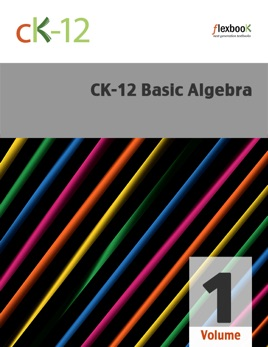## Publisher Description

CK-12 Foundation's Basic Algebra, Volume 1 FlexBook covers the following six chapters:

Expressions, Equations, and Functions - covers the relationships among expressions, equations, and functions when variables are present. Also explored is how these ideas can be shown on graphs. Properties of Real Numbers - covers various forms that rational numbers can assume, including fractions, integers, and square roots. Also considered are different operations for manipulating rational numbers. Linear Equations - introduces students to methods of solving simple equations involving variables. Also covered are ratios, scale, and the percent equation. Graphing Linear Equations and Functions - provides students with a more in-depth understanding of equations by introducing coordinate plane graphing concepts such as intercepts and slope. Writing Linear Equations - focuses on writing various forms of equations based upon real-world data and already existing lines. Students will also learn about predicting data using a fitted line. Linear Inequalities and Absolute Value; An Introduction to Probability - covers operations of inequalities, including addition, subtraction, multiplication and division. These principles are then applied to absolute value and probability.

## Customer Reviews

jch1939 ,

### Too Many Bugs

Good subject exposition but there are too many bugs with text handling and none of the videos play in Safari. No interactive problem solving.

Not quite ready for prime time.

TayTay 2003 ,

### Ck -12 algebra

I've only read a little bit of the book.But I think It will help me In school.

Three Leg Bambi ,

### Huge gaps in text.

None of the equations or formulas are displayed. It says to solve,
,using . Thats it. A whole mess of blanks. Thanks. This wont help me whatsoever. Please fix.